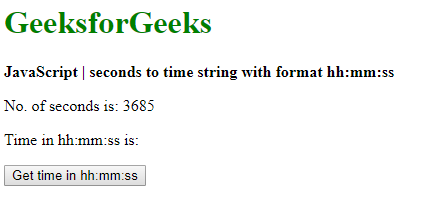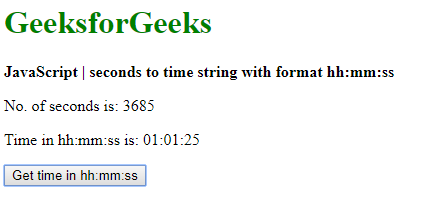# How to convert seconds to time string format hh:mm:ss using JavaScript ?

Given a time in seconds and the task is to convert the time into a string format hh:mm:ss. There are two approaches to solve this problem:

Method 1: Passing the seconds to a date object

• The Date() constructor expects a UNIX timestamp as one of its forms. A UNIX timestamp is a number of milliseconds that have passed since the epoch time (January 1, 1970, 00:00:00 UTC). The number of seconds can be given to this constructor after converting it to milliseconds.
• The time in seconds is converted to a time in milliseconds by multiplying it by 1000. This is passed to the Date() constructor. The Date object created can then be used to access the hours, minutes and seconds as required for the format.
• The hours value is extracted from the date using the getUTCHours() method. The minutes value in UTC is extracted from the date using the getUTCMinutes() method. The seconds value is extracted from the date using the getSeconds() method.
• The final formatted date is created by converting each of these values to a string using the toString() method and then padding them with an extra ‘0’, if the value is a single-digit by using the padStart() method. The individual parts are then joined together with a colon(:) as the separator. This string is in the required format “hh:mm:ss”.

Syntax:

```dateObj = new Date(given_seconds * 1000);
hours = dateObj.getUTCHours();
minutes = dateObj.getUTCMinutes();
seconds = dateObj.getSeconds();

timeString = hours.toString().padStart(2, '0') + ':' +
```

Example:

 ` ` `<``html``> ` ` `  `<``head``> ` `    ``<``title``> ` `        ``How to convert seconds to time string ` `        ``format hh:mm:ss using JavaScript ? ` `    `` ` ` ` ` `  `<``body``> ` `    ``<``h1` `style``=``"color: green"``> ` `        ``GeeksforGeeks ` `    `` ` `     `  `    ``<``b``> ` `        ``JavaScript | seconds to time ` `        ``string with format hh:mm:ss ` `    `` ` `     `  `    ``<``p``>No. of secomds is: 3685 ` `     `  `    ``<``p``> ` `        ``Time in hh:mm:ss is: ` `        ``<``span` `class``=``"output"``> ` `    `` ` ` `  `    ``<``button` `onclick``=``"convertSecondstoTime()"``> ` `        ``Get time in hh:mm:ss ` `    `` ` `     `  `    ``<``script` `type``=``"text/javascript"``> ` `        ``function convertSecondstoTime() { ` `            ``given_seconds = 3685; ` ` `  `            ``dateObj = new Date(given_seconds * 1000); ` `            ``hours = dateObj.getUTCHours(); ` `            ``minutes = dateObj.getUTCMinutes(); ` `            ``seconds = dateObj.getSeconds(); ` ` `  `            ``timeString = hours.toString().padStart(2, '0') ` `                ``+ ':' + minutes.toString().padStart(2, '0') ` `                ``+ ':' + seconds.toString().padStart(2, '0'); ` ` `  `            ``document.querySelector('.output').textContent ` `                        ``= timeString; ` `        ``} ` `    `` ` ` ` ` `  ` `

Output:

• Before clicking the button:• After clicking the button:Method 2: Calculating the hours, minute and seconds individually

• The hours can be calculated by dividing the seconds by 3600 since one hour equals 3600 seconds. This will find out the number of hours. This number is brought down to the nearest integer by using the Math.floor() function.
• The minutes can be calculated by finding the number of seconds left after subtracting the number of hours. This value is divided by 60 to get the number of minutes. This number is brought down to the nearest integer by using the Math.floor() function.
• The seconds can be calculated by subtracting the number of seconds in the minutes value and the number of seconds in the hours value from the total seconds given before.
• The final formatted date is created by converting each of these values to a string using the toString() method and then padding them with an extra ‘0’, if the value is a single-digit by using the padStart() method. The individual parts are then joined together with a colon(:) as the separator. This string is in the required format “hh:mm:ss”.

Syntax:

```hours = Math.floor(given_seconds / 3600);
minutes = Math.floor((given_seconds - (hours * 3600)) / 60);
seconds = given_seconds - (hours * 3600) - (minutes * 60);

timeString = hours.toString().padStart(2, '0') + ':' +
```

Example:

 `                        `` ` `<``html``> ` ` `  `<``head``> ` `    ``<``title``> ` `        ``How to convert seconds to time string ` `        ``format hh:mm:ss using JavaScript ? ` `    `` ` ` ` ` `  `<``body``> ` `    ``<``h1` `style``=``"color: green"``> ` `        ``GeeksforGeeks ` `    `` ` `     `  `    ``<``b``> ` `        ``JavaScript | seconds to time  ` `        ``string with format hh:mm:ss ` `    `` ` `     `  `    ``<``p``>No. of secomds is: 3685 ` `    ``<``p``> ` `        ``Time in hh:mm:ss is:  ` `        ``<``span` `class``=``"output"``> ` `    `` ` ` `  `    ``<``button` `onclick``=``"convertSecondstoTime()"``> ` `        ``Get time in hh:mm:ss ` `    `` ` `     `  `    ``<``script` `type``=``"text/javascript"``> ` `        ``function convertSecondstoTime() { ` `            ``given_seconds = 3685; ` ` `  `            ``hours = Math.floor(given_seconds / 3600); ` `            ``minutes = Math.floor((given_seconds - (hours * 3600)) / 60); ` `            ``seconds = given_seconds - (hours * 3600) - (minutes * 60); ` ` `  `            ``timeString = hours.toString().padStart(2, '0') + ':' + ` `                ``minutes.toString().padStart(2, '0') + ':' + ` `                ``seconds.toString().padStart(2, '0'); ` ` `  `            ``document.querySelector('.output').textContent ` `                        ``= timeString; ` `        ``} ` `    `` ` ` ` ` `  ` `

Output:

• Before clicking the button:• After clicking the button:My Personal Notes arrow_drop_upCheck out this Author's contributed articles.

If you like GeeksforGeeks and would like to contribute, you can also write an article using contribute.geeksforgeeks.org or mail your article to contribute@geeksforgeeks.org. See your article appearing on the GeeksforGeeks main page and help other Geeks.

Please Improve this article if you find anything incorrect by clicking on the "Improve Article" button below.